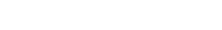# Double Major | Mathematics | SIU

HomeCONTACT

SIU.EDU

### College of Engineering, Computing, Technology, and Mathematics## Double Major

Special provisions are made for students to earn a double major in mathematics and a field in which mathematics is extensively applied. The courses Math 447, 449, 471, 472 and 475 carry credit in both mathematics and computer science. See Bachelor of Science Degree, College of Science for specific requirements in mathematics for students who also earn a major or minor in computer science.

For students pursuing a double major in math and engineering, physics, or chemistry, the mathematics requirements are Math 150, 250, 251, 305 and five additional mathematics courses numbered above 300, including at least three courses above 400, and including two of the three areas of algebra, analysis, probability and statistics. A mathematics department advisor must approve the courses.

Students majoring in business and administration with a secondary concentration in mathematics may obtain a second major in mathematics. The requirements are Mathematics 150, 221, 250, 251 and five approved mathematics courses at the 300-400 level, of which at least four are at the 400-level. Recommended courses for this program include Mathematics 471, 472, 475A, 483, 484, Management 352, 360, 456; Economics 315, 465; Finance 310, 331 and 341.

A grade of C or better is required in every mathematics course used to satisfy departmental requirements.

### Double majors in Engineering, Physics, or Chemistry

• Math 150, 221, 250, 251, 305
• 5 additional mathematics courses numbered above 300.  These must include at least 3 courses above 400, and must cover 2 of groups A (Algebra/Discrete Math), B (Analysis), and D (Probability/Statistics) (see below).
• The courses must be approved by a mathematics department advisor.

Group A: Algebra/Discrete Math/Linear Algebra: 319, 349, 421
Group B: Analysis: 352, 450, 452, 455
Group C: Applied Math/Numerical Analysis: 305, 471, 472, 475a
Group D: Probability/Statistics: 380, 480, 483

### Double majors in business with a secondary concentration in mathematics

• Math 150, 221, 250, 251
• 5 mathematics courses at the 300-400 level, of which at least 4 are at the 400 level.  Recommended courses are Math 471, 472, 475, 483, 484
• The courses must be approved by a mathematics department advisor.